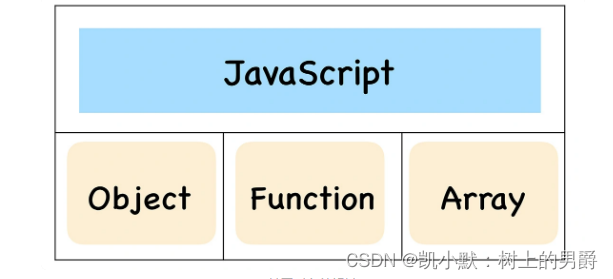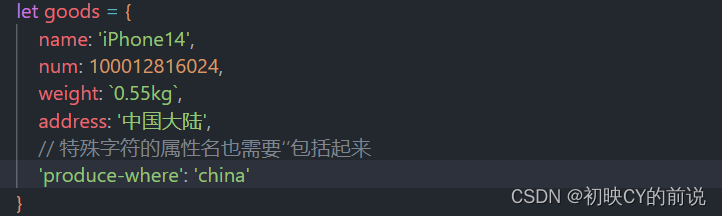# js Map对象的用法

+关注继续查看

## 一、Map对象方法

Map：

### Map是一组键值对的结构，具有极快的查找速度。

var names = ['Michael', 'Bob', 'Tracy'];
var scores = [95, 75, 85];

var m = new Map([['Michael', 95], ['Bob', 75], ['Tracy', 85]]);
m.get('Michael'); // 95

var m = new Map(); // 空Map
m.set('Bob', 59);
m.get('Adam'); // undefined

var m = new Map();
m.get('Adam'); // 88

• Set：

Set和Map类似，也是一组key的集合，但不存储value。由于key不能重复，所以，在Set中，没有重复的key。

var s1 = new Set(); // 空Set
var s2 = new Set([1, 2, 3]); // 含1, 2, 3

var s = new Set([1, 2, 3, 3, '3']);
s; // Set {1, 2, 3, "3"}

s.add(4);
s; // Set {1, 2, 3, 4}
s; // 仍然是 Set {1, 2, 3, 4}

var s = new Set([1, 2, 3]);
s; // Set {1, 2, 3}
s.delete(3);
s; // Set {1, 2}

## 二、定义：键/值对的集合。

• 语法：mapObj = new Map() 备注：集合中的键和值可以是任何类型。如果使用现有密钥向集合添加值，则新值会替换旧值。

构造函数
指定创建映射的函数。
Prototype — 原型
为映射返回对原型的引用。
size
返回映射中的元素数。

clear
从映射中移除所有元素。
delete
从映射中移除指定的元素。
forEach
对映射中的每个元素执行指定操作。
get
返回映射中的指定元素。
has
如果映射包含指定元素，则返回 true。
set
添加一个新建元素到映射。
toString
返回映射的字符串表示形式。
valueOf
返回指定对象的原始值。

var m = new Map();
m.set(1, "black");
m.set(2, "red");
m.set("colors", 2);
m.set({x:1}, 3);
m.forEach(function (item, key, mapObj) {
document.write(item.toString() + "<br />");
});
document.write("<br />");
document.write(m.get(2));
// Output:
// black
// red
// 2
// 3
//
// red

## 三、ES6入门

ES6的Set和Map数据结构 ：

Set和Map主要的应用场景在于数组去重和数据存储，

Set是一种叫做集合的数据结构，Map是一种叫做字典的数据结构

const s = new Set();

[2, 3, 5, 4, 5, 2, 2].forEach(x => s.add(x));

for (let i of s) {
console.log(i);   // 2 3 5 4
}

### 去除数组的重复成员

let array = [1,2,1,4,5,3];
[...new Set(array)]     // [1, 2, 4, 5, 3]

### Set实例的属性和方法

Set的属性： size：返回集合所包含元素的数量 Set的方法： 操作方法

add(value)：向集合添加一个新的项
delete(value)：从集合中移除一个值
has(value)：如果值在集合中存在，返回true,否则false
clear(): 移除集合里所有的项

keys()：返回一个包含集合中所有键的数组
values()：返回一个包含集合中所有值的数组
entries：返回一个包含集合中所有键值对的数组(感觉没什么用就不实现了)
forEach()：用于对集合成员执行某种操作，没有返回值

function Set(arr = []) {    // 可以传入数组
let items = {};
this.size = 0;  // 记录集合中成员的数量
}

module.exports = Set;

#### has方法

function Set() {
let items = {};
this.size = 0;

// has(val)方法
this.has = function(val) {
// 对象都有hasOwnProperty方法，判断是否拥有特定属性
return items.hasOwnProperty(val);
};
}

// add(val)方法
if (!this.has(val)) {
items[val] = val;
this.size++;    // 累加集合成员数量
return true;
}
return false;
};

#### delete和clear方法

// delete(val)方法
this.delete = function(val) {
if (this.has(val)) {
delete items[val];  // 将items对象上的属性删掉
this.size--;
return true;
}
return false;
};
// clear方法
this.clear = function() {
items = {};     // 直接将集合赋一个空对象即可
this.size = 0;
};

#### keys、values方法

// keys()方法
this.keys = function() {
return Object.keys(items);  // 返回遍历集合的所有键名的数组
};
// values()方法
this.values = function() {
return Object.values(items);  // 返回遍历集合的所有键值的数组
};

// set.js
const Set = require('./Set.js');    // 导入写好的Set类
let set = new Set();
console.log(set.keys());    // [ '1', '2', '3' ]
console.log(set.values());  // [ 1, 2, 3 ]

#### forEach方法

ES6中Set结构的实例上带的forEach方法，其实和数组的forEach方法很相似，只不过Set结构的键名就是键值，所以第一个参数与第二个参数的值永远都是一样的

// forEach(fn, context)方法
this.forEach = function(fn, context = this) {
for (let i = 0; i < this.size; i++) {
let item = Object.keys(items)[i];
fn.call(context, item, item, items);
}
};


// set.js
const Set = require('./Set.js');
let set = new Set();
set.forEach((value, key) => console.log(key + ' : ' + value));  // 1:1, 3:3, 4:4
let arr = set.values();     // [ 1, 3, 4 ]
arr = new Set(arr.map(x => x * 2)).values();
console.log(arr);           // [ 2, 6, 8 ]

function Set(arr = []) {    // 传入接受的数组，如果没有传指定一个空数组做为初始值
let items = {};
this.size = 0;
// has方法
this.has = function (val) {
return items.hasOwnProperty(val);
};
// 如果没有存在items里面就可以直接写入
if (!this.has(val)) {
items[val] = val;
this.size++;
return true;
}
return false;
};
arr.forEach((val, i) => {   // 遍历传入的数组
});
// 省略...
}

// 间接使用map和filter
const Set = require('./Set.js');
let arr = new Set([1, 2, 3]).values();
m = new Set(arr.map(x => x * 2));
f = new Set(arr.filter(x => x>1));
console.log(m.values());    // [ 2, 4, 6 ]
console.log(f.values());    // [ 2, 3 ]

// 数组去重
let arr2 = new Set([3, 5, 2, 1, 2, 5, 5]).values();
console.log(arr2);  // [ 1, 2, 3, 5 ]

#### union并集和intersect交集

// 并集
this.union = function (other) {
let union = new Set();
let values = this.values();
for (let i = 0; i < values.length; i++) {
}
values = other.values();    // 将values重新赋值为新的集合
for (let i = 0; i < values.length; i++) {
}

return union;
};
// 交集
this.intersect = function (other) {
let intersect = new Set();
let values = this.values();
for (let i = 0; i < values.length; i++) {
if (other.has(values[i])) {     // 查看是否也存在于other中
}
}
return intersect;
};

#### difference差集

// 差集
this.difference = function (other) {
let difference = new Set();
let values = this.values();
for (let i = 0; i < values.length; i++) {
if (!other.has(values[i])) {    // 将不存在于other集合中的添加到新的集合中
}
}
return difference;
};

• Set完整实现

function Set(arr = []) {
let items = {};
this.size = 0;
// has方法
this.has = function (val) {
return items.hasOwnProperty(val);
};
// 如果没有存在items里面就可以直接写入
if (!this.has(val)) {
items[val] = val;
this.size++;
return true;
}
return false;
};
arr.forEach((val, i) => {
});
// delete方法
this.delete = function (val) {
if (this.has(val)) {
delete items[val];  // 将items对象上的属性删掉
this.size--;
return true;
}
return false;
};
// clear方法
this.clear = function () {
items = {};
this.size = 0;
};
// keys方法
this.keys = function () {
return Object.keys(items);
};
// values方法
this.values = function () {
return Object.values(items);
}
// forEach方法
this.forEach = function (fn, context = this) {
for (let i = 0; i < this.size; i++) {
let item = Object.keys(items)[i];
fn.call(context, item, item, items);
}
}

// 并集
this.union = function (other) {
let union = new Set();
let values = this.values();

for (let i = 0; i < values.length; i++) {
}
values = other.values();    // 将values重新赋值为新的集合
for (let i = 0; i < values.length; i++) {
}

return union;
};
// 交集
this.intersect = function (other) {
let intersect = new Set();
let values = this.values();
for (let i = 0; i < values.length; i++) {
if (other.has(values[i])) {
}
}
return intersect;
};
// 差集
this.difference = function (other) {
let difference = new Set();
let values = this.values();
for (let i = 0; i < values.length; i++) {
if (!other.has(values[i])) {
}
}
return difference;
};
// 子集
this.subset = function(other) {
if (this.size > other.size) {
return false;
} else {
let values = this.values();
for (let i = 0; i < values.length; i++) {
console.log(values[i])
console.log(other.values())
if (!other.has(values[i])) {
return false;
}
}
return true;
}
};
}

module.exports = Set;

const Set = require('./Set.js');
let set = new Set([2, 1, 3]);
console.log(set.keys());    // [ '1', '2', '3' ]
console.log(set.values());  // [ 1, 2, 3 ]
console.log(set.size);      // 3
set.delete(1);
console.log(set.values());  // [ 2, 3 ]
set.clear();
console.log(set.size);      // 0

// 并集
let a = [1, 2, 3];
let b = new Set([4, 3, 2]);
let union = new Set(a).union(b).values();
console.log(union);     // [ 1, 2, 3, 4 ]

// 交集
let c = new Set([4, 3, 2]);
let intersect = new Set([1,2,3]).intersect(c).values();
console.log(intersect); // [ 2, 3 ]

// 差集
let d = new Set([4, 3, 2]);
let difference = new Set([1,2,3]).difference(d).values();
// [1,2,3]和[4,3,2]的差集是1
console.log(difference);    // [ 1 ]

#### 字典

const m = new Map();
const o = {p: 'Hello World'};
m.set(o, 'content')
m.get(o) // "content"

m.has(o) // true
m.delete(o) // true
m.has(o) // false

• Map的属性和方法

• size：返回字典所包含的元素个数 操作方法：

set(key, val): 向字典中添加新元素
get(key):通过键值查找特定的数值并返回
has(key):如果键存在字典中返回true,否则false
delete(key): 通过键值从字典中移除对应的数据
clear():将这个字典中的所有元素删除

keys():将字典中包含的所有键名以数组形式返回
values():将字典中包含的所有数值以数组形式返回
forEach()：遍历字典的所有成员

function Map() {
let items = {};
}

module.exports = Map;   // 导出

• has方法

function Map() {
let items = {};
// has(key)方法
this.has = function(val) {
return items.hasOwnProperty(val);
};
}

• set和get方法

// set(key, val)方法
// set相同key时，后面声明的会覆盖前面
// 如： new Map().set({}, 'a')
this.set = function(key, val) {
items[key] = val;
};
// get(key)方法
this.get = function(key) {
// 判断是否有key，如果有的话直接返回对应的值
// 如果读取一个未知的键，则返回undefined
return this.has(key) ? items[key] : undefined;
};

set和get方法写好了，再接着搞delete和clear方法，不废话，看
delete和clear方法
// delete(key)方法
this.delete = function(key) {
if (this.has(key)) {    // 如果有key值
delete items[key];  // 直接删掉items上对应的属性
this.size--;        // 让size总数减1
return true;
}
return false;
};
// clear()方法
this.clear = function() {
items = {};
this.size = 0;
};

// keys()方法
this.keys = function() {
return Object.keys(items);
};
// values()方法
this.values = function() {
return Object.values(items);
};
// forEach(fn, context)方法
this.forEach = function(fn, context = this) {
for (let i = 0; i < this.size; i++) {
let key = Object.keys(items)[i];
let value = Object.values(items)[i];
fn.call(context, value, key, items);
}
};

Map完整实现

function Map() {
let items = {};
this.size = 0;

// 操作方法
// has方法
this.has = function(val) {
return items.hasOwnProperty(val);
};
// set(key, val)方法
this.set = function(key, val) {
items[key] = val;
this.size++;
};
// get(key)方法
this.get = function(key) {
return this.has(key) ? items[key] : undefined;
};
// delete(key)方法
this.delete = function(key) {
if (this.has(key)) {
delete items[key];
this.size--;
return true;
}
return false;
};
// clear()方法
this.clear = function() {
items = {};
this.size = 0;
};
// 遍历方法
// keys()方法
this.keys = function() {
return Object.keys(items);
};
// values()方法
this.values = function() {
return Object.values(items);
};
// forEach(fn, context)方法
this.forEach = function(fn, context = this) {
for (let i = 0; i < this.size; i++) {
let key = Object.keys(items)[i];
let value = Object.values(items)[i];
fn.call(context, value, key, items);
}
};
}

module.exports = Map;

// map.js
// 使用Map类
const Map = require('./Map.js');
let m = new Map();
m.set('Jay', 'Jay的Chou');
m.set(true, '真的');
console.log(m.has('Chou'));  // false
console.log(m.size);        // 2
console.log(m.keys());      // [ 'Jay', 'true' ]
console.log(m.values());    // [ 'Jay的Chou', '真的' ]
console.log(m.get('jay'));  // undefined

m.delete(true);
console.log(m.keys());      // [ 'Jay' ]
console.log(m.values());    // [ 'Jay的Chou' ]

### 四、ES6为Array增加了from函数用来将其他对象转换成数组。

#### 2.类数组对象，什么叫类数组对象，就是一个对象必须有length属性，没有length，转出来的就是空数组。

• 转换map

const map1 = new Map();
map1.set('k1', 1);
map1.set('k2', 2);
map1.set('k3', 3);
console.log('%s', Array.from(map1))

k1,1,k2,2,k3,3

• 转换set

const set1 = new Set();
console.log('%s', Array.from(set1))

1,2,3

• 转换字符串

console.log('%s', Array.from('hello world'))
console.log('%s', Array.from('\u767d\u8272\u7684\u6d77'))

h,e,l,l,o, ,w,o,r,l,d

• 类数组对象

console.log('%s', Array.from({
0: '0',
1: '1',
3: '3',
length:4
}))

0,1,,3

console.log('%s', Array.from({
0: 0,
1: 1
}))

console.log('%s', Array.from({
a: '1',
b: '2',
length:2
}))

Array.from可以接受三个参数

Array.from(arrayLike[, mapFn[, thisArg]])
arrayLike：被转换的的对象。
mapFn：map函数。
thisArg：map函数中this指向的对象。

console.log('%s', Array.from([1, 2, 3, 4, 5], (n) => n + 1))

2,3,4,5,6

let diObj = {
handle: function(n){
return n + 2
}
}
console.log('%s', Array.from(
[1, 2, 3, 4, 5],
function (x){
return this.handle(x)
},
diObj))

3,4,5,6,7JavaScript Math 对象允许您对数字执行数学任务。
JavaScript Math 对象允许您对数字执行数学任务。
6 0JavaScript的对象有什么作用？底层原理是什么？
JavaScript的对象有什么作用？底层原理是什么？
10 019 0JavaScript 中的对象
JavaScript 中的对象
17 0JavaScript 中的所有事物都是对象
JavaScript 中的所有事物都是对象
20 0JavaScript对象必会的基操（ES6以前）

19 0JavaScript对象高级 ③
JavaScript对象高级 ③
26 0js 把字符串转成json对象的三种方法
js 把字符串转成json对象的三种方法
32 020 0javaScript 04 对象的使用

18 0

JavaScript 自学手册文档教程323765

JavaScript入门与实战1923452

Node.js 入门教程文档394825

Node.js 入门与实战1322489

Javascript中的函数

Javascript异步编程

JS零基础入门教程（上册）